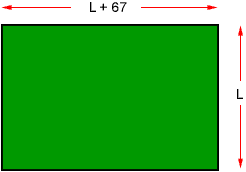Hi, Can you please help my daughter solve this Algebra, 9th grade, problem. She needs to write a expresion for the following, The width of a football field is 67ft greater than the length. The perimeter is 480ft What is the width and length? Thank you Paige Hi Paige, First, are you sure that you have the problem worded correctly? You have described a football field that is wider than it is long. Anyway, the first thing I would do is to draw a diagram. In the diagram I let L be the length of the field in feet.You also know that the perimeter, the total length around the outside, is 480 feet. Imagine that you start at the upper left corner of the field and go around the field clockwise, measuring the perimeter. You get (L + 67)+ L + (L + 67) + L This is the perimeter, so (L + 67)+ L + (L + 67) + L = 480 Now solve for L. Cheers, Penny Go to Math Central